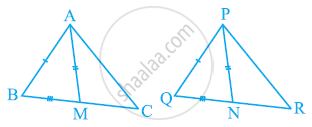# Two sides AB and BC and median AM of one triangle ABC are respectively equal to sides PQ and QR and median PN of ΔPQR (see the given figure). Show that: (i) ΔABM ≅ ΔPQN (ii) ΔABC ≅ ΔPQR - Mathematics

Two sides AB and BC and median AM of one triangle ABC are respectively equal to sides PQ and QR and median PN of ΔPQR (see the given figure). Show that:

(i) ΔABM ≅ ΔPQN

(ii) ΔABC ≅ ΔPQR#### Solution

(i) In ΔABC, AM is the median to BC.

∴ BM = 1/2BC

In ΔPQR, PN is the median to QR.

∴ QN = 1/2QR

However, BC = QR

∴ 1/2BC = 1/2QR

⇒ BM = QN … (1)

In ΔABM and ΔPQN,

AB = PQ (Given)

BM = QN [From equation (1)]

AM = PN (Given)

∴ ΔABM ≅ ΔPQN (SSS congruence rule)

∠ABM = ∠PQN (By CPCT)

∠ABC = ∠PQR … (2)

(ii) In ΔABC and ΔPQR,

AB = PQ (Given)

∠ABC = ∠PQR [From equation (2)]

BC = QR (Given)

⇒ ΔABC ≅ ΔPQR (By SAS congruence rule)

Concept: Some More Criteria for Congruence of Triangles
Is there an error in this question or solution?

#### APPEARS IN

NCERT Class 9 Maths
Chapter 7 Triangles
Exercise 7.3 | Q 3 | Page 128